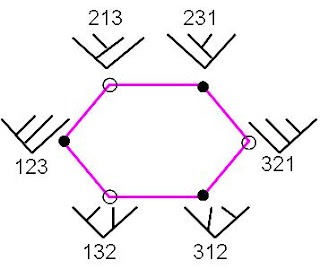## M Theory RevisionWhile Carl Brannen is on the subject of 1-circulants, let us recall the simple hexagon with both tree labels and the corresponding permutations from \$S_3\$. Note that the black nodes naturally form a 2-circulant matrix

123
231
312

whereas the open nodes form a 1-circulant

213
321
132

The first matrix contains the identity along with the other two permutations appearing in Bilson-Thompson’s ribbon diagrams. Such triple node sets must be idempotent if viewed as sources (or targets) since the source of a source (identity arrow) is just the source again. In ordinary 1-categories sources are usually single objects, but in 3-logos theory (or in looking at (co)operads as multicategories) they should be triples. If we add a central origin to the hexagon, turning it into a cube, the three black nodes could mark the three directions in Space. This puts a little cube at the target of the big cube, and Time is just what we see in a mirror.

## 6 Responses so far »

1. 1### CarlBrannen said,

Could you remind me, what’s the difference between a 1-circulant and a 2-circulant?

2. 2### Kea said,

Hi Carl. The 1-c has a right shift of 1 place per row, and the 2-c has a right shift of 2. Thus for nxn matrices there are 1,2,3,…,n-1 kinds of circulant. They interact in interesting ways: 1.1=1 , 1.2=2 , 2.1=2 and 2.2=1.

3. 3### Doug said,

Hi Kea,

I do not have any understanding of this type of notation, so I have two dumb questions for possible “alternative perspectives”.

1 – When I first saw your diagram, I was thinking that perhaps the black nodes represented real and the open nodes “imaginary” dimensions in complex space?

2 – Although the direction of circulation is probably clockwise by definition, is a counterclockwise circulation possible?

4. 4### Kea said,

Hi Doug. The real/imaginary division is indeed an interesting interpretation, and one could define the circuit in either direction.

5. 5### CarlBrannen said,

Hmmm. So the 2-circulants are square roots of 1-circulants, at least for 3×3 matrices.

The square of a 2-circulant is a restricted type of 1-circulant, the off diagonal elements are all equal. In the Koide formulas, that would be a system with a “that damned angle” of zero.

Interestingly, if you attribute “that damned angle” to soft bosons, then the electrons do have an angle of zero. They would be consistent with being the square of a 2-circulant.

But the neutrinos do have a geometric angle, pi/12.

6. 6### Kea said,

…then the electrons do have an angle of zero. They would be consistent with being the square of a 2-circulant.

Good points, Carl. Yes, I can’t help thinking the zero angle circulants are natural basis elements for something…. see this old reference.